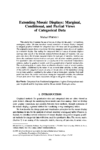Michael Friendly's

# Extending Mosaic Displays: Marginal, Conditional, and Partial Views of Categorical Data• Extending Mosaic Displays: Marginal, Conditional, and Partial Views of Categorical Data.
• Journal of Computational and Graphical Statistics, vol. 8, no. 3, pp. 373–395, 1999.

This article first illustrates the use of mosaic displays for the analysis of multiway contingency tables. We then introduce several extensions of mosaic displays designed to integrate graphical methods for categorical data with those used for quantitative data. The scatterplot matrix shows all painvise (bivariate marginal) views of a set of variables in a coherent display. One analog for categorical data is a matrix of mosaic displays showing some aspect of the bivariate relation between all pairs of variables. The simplest case shows the bivariate marginal relation for each pair of variables. Another case shows the conditional relation between each pair, with all other variables partialled out. For quantitative data this represents (a) a visualization of the conditional independence relations studied by graphical models, and (b) a generalization of partial residual plots. The conditioning plot, or coplot, shows a collection of partial views of several quantitative variables, conditioned by the values of one or more other variables. A direct analog of the coplot for categorical data is an array of mosaic plots of the dependence among two or more variables, stratified by the values of one or more given variables. Each such panel then shows the partial associations among the foreground variables; the collection of such plots shows how these associations change as the given variables vary.

```@Article{Friendly99EMD,
author = {Michael Friendly},
year = {1999},
title = {Extending Mosaic Displays: Marginal, Conditional, and Partial Views of Categorical
Data},
journal = {Journal of Computational and Graphical Statistics},
volume = {8},
number = {3},
pages = {373–395},
url = {http://datavis.ca/papers/jcgs-extending.pdf},
doi = {10.1080/10618600.1999.10474820},
}```